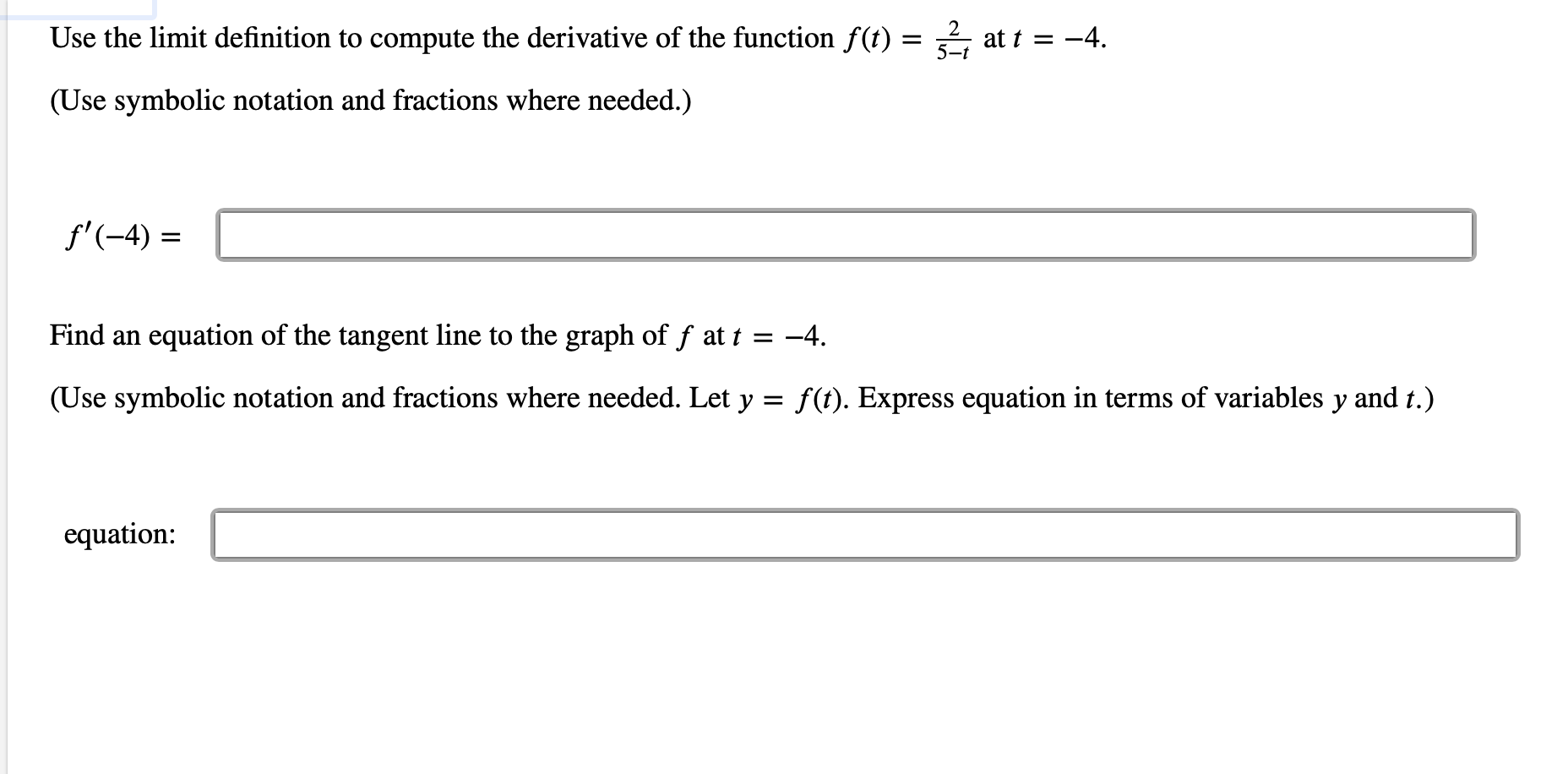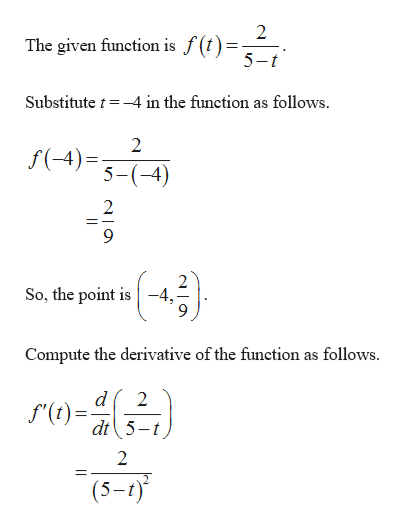# Use the limit definition to compute the derivative of the function f(t):5-tat t -4= _(Use symbolic notation and fractions where needed.)f'-4)Find anequation of the tangent line to the graph of f at t =-4.(Use symbolic notation and fractions where needed. Let y = f(t). Express equation in terms of variables y and t.)equation:

Questionhelp_outlineImage TranscriptioncloseUse the limit definition to compute the derivative of the function f(t): 5-t at t -4 = _ (Use symbolic notation and fractions where needed.) f'-4) Find an equation of the tangent line to the graph of f at t = -4. (Use symbolic notation and fractions where needed. Let y = f(t). Express equation in terms of variables y and t.) equation: fullscreen
check_circleExpert Solution
Step 1

Consider the given funct...help_outlineImage Transcriptionclose2 The given function is J 5-t Substitute t-4 in the function as follows 2 f(4)= 5-(-4) 2 2 So, the point is-4 Compute the derivative of the function as follows d f'(t) dt5-t 2 2 (5-) fullscreen

### Want to see the full answer?

See Solution

#### Want to see this answer and more?

Solutions are written by subject experts who are available 24/7. Questions are typically answered within 1 hour*

See Solution
*Response times may vary by subject and question
Tagged in

### Other